GFG App
Open AppBrowser
Continue

# Resistors in Series and Parallel Combinations

Resistors are devices which control the flow of electric current in the circuit. They provide the hindrance to the path of the current which flows in the circuit. Resistors consume the current in any circuit and convert them to other forms of energy as required. Various resistors can be added to the circuits as per our requirements. Two or more resistors can easily be added in particular sequences. The addition of resistors can be achieved using any of the two methods i.e. Series addition and parallel addition. In this article, we will learn about the addition of Resistors in Series and Parallel combinations and other in detail.

## Components of a Circuit

An electric circuit is made up of various components, some of the various components of the electric circuit are,

• Conductors (wires)
• Power Source
• Switch

Generally, copper wires are used as a conductor. Switch are components that control the entry and exit of the current in the circuit. used to make or break a circuit. Resistors are used to control the flow of the electric current in a circuit. Loads are devices that are used in a circuit that consumes electrical energy and converts it into other forms of energy like light, heat, sound, etc. Examples of load include bulbs, speakers, heaters, etc.

## Need for a Combination Circuit

In an electric circuit, resistive networks are made by combining various components of circuits together. They can be connected in two ways either in series combination or in parallel combination or in both, i.e. in any electric circuit, we can use both combinations also. These combinations are made to form various complex circuits which are then used for various purposes. We have to make sure that in end we must know the total resistance of the circuit to solve our problem.

## Resistors and Resistance

Resistors are electrical devices that restrict the flow of current in a circuit. It is an ohmic device, which means that it follows the ohms’ law V = IR. Most of the circuits have only one resistor, but sometimes more than one resistor can be present in the circuit. In that case, the current flowing through the circuit depends on the equivalent resistance of the combination. These combinations can be arbitrarily complex, but they can be divided into two basic types:

• Series Combination
• Parallel Combination

## Resistors in Series

In the figure given below, three resistors are connected in series with the battery of voltage V. In this type of combination, resistors are usually connected in a sequential manner one after another. The current through each resistor is the same. The figure on the right side shows the equivalent resistance of the three resistances. In the case of the series combination of resistances, the equivalent resistance is given by the algebraic sum of the individual resistances. The diagram given below shows the resistor added in a series combination.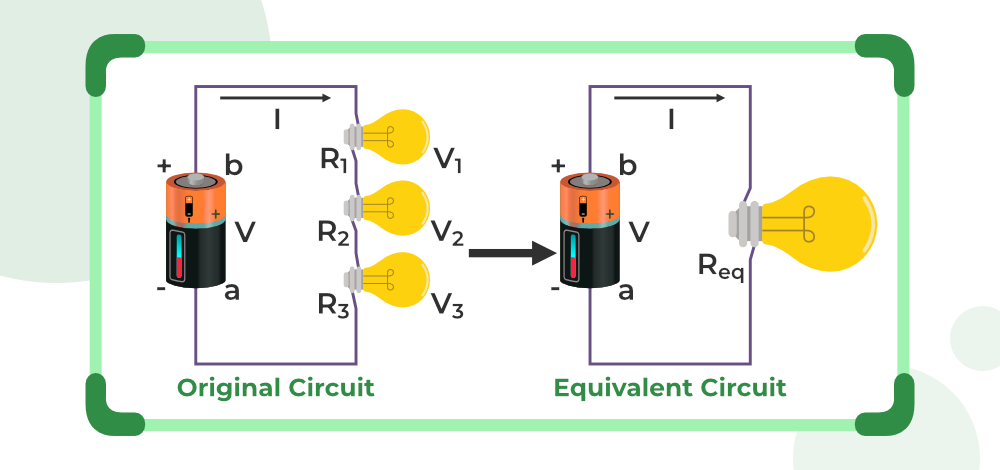Let V1, V2, and V3 be the voltages across all three resistances. It is known that the current flowing through them is the same.

V = V + V2 + V3

Expanding the equation,

IR = IR + IR2 + IR3

IR = I(R1 + R2 + R3)

R = R1 + R2 + R3

## Resistors in Parallel

In the figure given below, three resistors are shown which are connected in parallel with a battery of voltage V. In this type of connection, the resistors are usually connected on parallel wires originating from a common point. In this case, the voltage through each resistor is the same. The figure on the right side shows the equivalent resistance of the three resistances. The diagram given below shows the resistor added in parallel combination.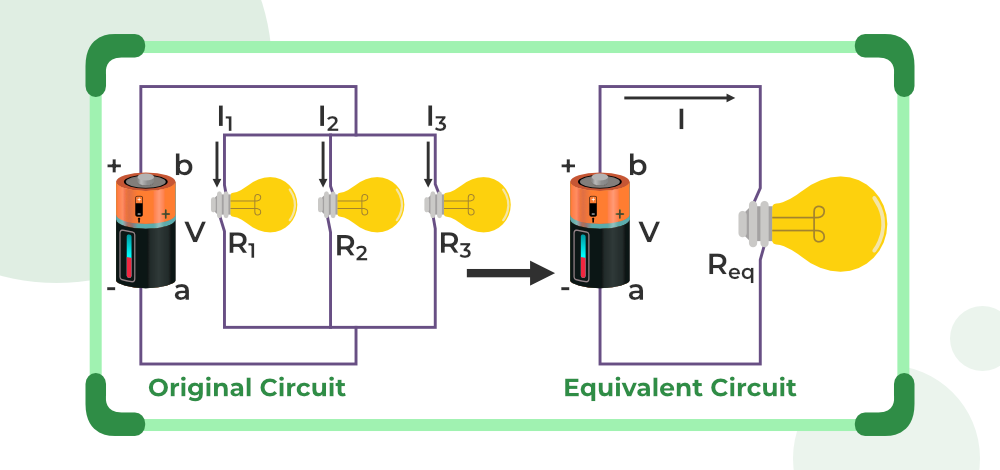The relation between voltage and current is given by,

V = IR

It can be rewritten as,

I = V/R

The voltages across individual resistors will be,

I1 = V/R1 , I2 = V/R2, I3 = V/R3

The total current across all the resistances will be,

I = I1 + I2 + I3

Substituting the expressions for individual voltages,

I = V/R1 + V/R + V/R3

Let the equivalent capacitance be R,

V/R = V/R1 +V/R + V/R3

Upon Simplifying the above equation, the relation becomes,In general for capacitors R1, R2, R3,…

### Equivalent Parallel Resistance## Points To Remember

Various points to remember from the above discussion include,

A circuit consists of conductors (wires), power sources, loads, resistors, and switches.

• The entry and exit of the current in the circuit are controlled by the switches.
• The flow of the electric current in the circuit is managed by the resistors.
• In a series combination of circuits same current flows in all the resistors. The total or equivalent resistance can be given by,

Rtotal = R1 + R2 + ….. + Rn

• In a parallel combination of circuits, the voltage is similar in all the resistors. The total or equivalent resistance can be given by,

1 / Rtotal = 1 / R1 + 1 / R2 + ….. + 1 / Rn

## Solved Examples on Resistors in Series and Parallel Combinations

Example 1: Three resistances of 3, 5, and 10 ohms are connected in series. Find the equivalent resistance for the system.

Solution:

The formula for series resistance is given by,

R = R1 + R2 + R3

Given: R1 = 3, R = 5 and R3 = 10

Substituting these values in the equation,

R = R1 + R2 + R3

R = 3 + 5 + 10

R = 18 Ω

Example 2: Three resistances of 2, 2, and 4 ohms are connected in parallel. Find the equivalent resistance for the system.

Solution:

The formula for parallel resistance is given by,Given: R1 = 2, R = 2 and R3 = 4

Substituting these values in the equation,Ω

Example 3: Find the equivalent resistance for the system shown in the figure below.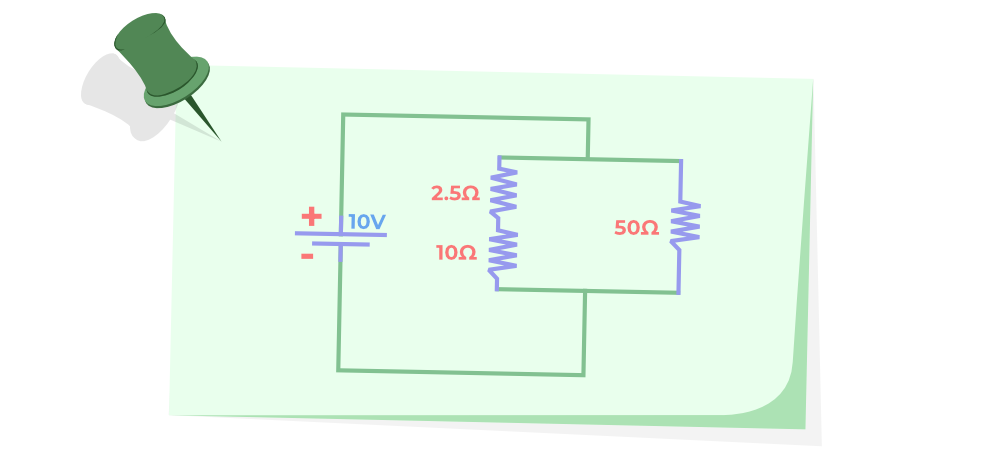Solution:

The formula for parallel resistance is given by,And the formula for series resistance is given by,

R = R1 + R2 + R + ….

This is combination of both parallel and series resistances.

Substituting these values in the equation,

R1 = 10 Ω, R2  = 2.5 Ω

R= R1 + R2

R = 10 + 2.5

R = 12.5R = 5 Ω

Example 4: Find the equivalent resistance for the system shown in the figure below.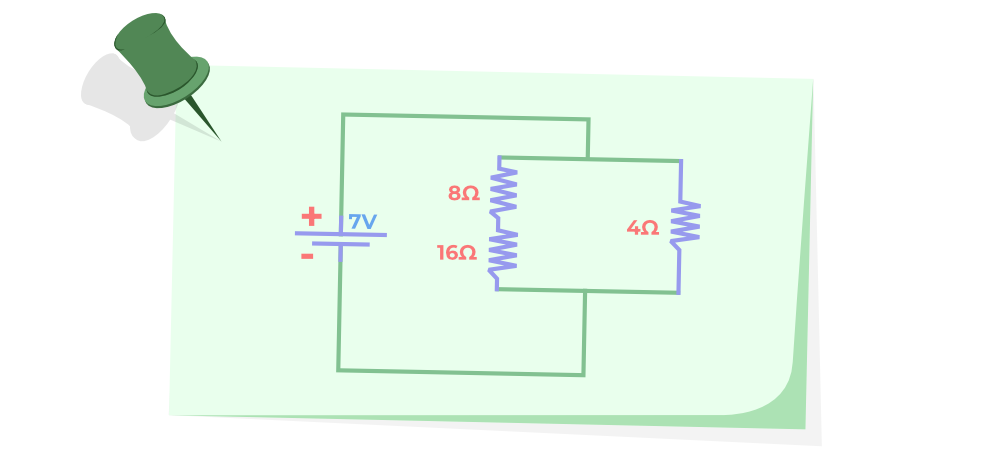Solution:

The formula for parallel resistance is given by,And the formula for series resistance is given by,

R = R1 + R2 + R + ….

This is combination of both parallel and series resistances.

Substituting these values in the equation,

R1 = 8 Ω, R2 = 16 Ω

R= R1 + R2

R = 8 + 16

R = 24R = 24 / 7 Ω

Example 5: Find the equivalent resistance for the system shown in the figure below.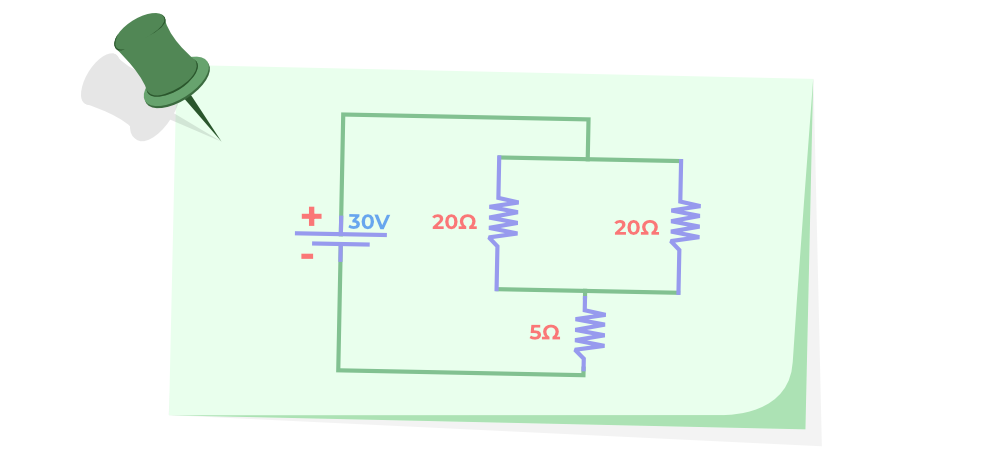Solution:

The formula for parallel resistance is given by,And the formula for series resistance is given by,

R = R1 + R2 + R + …

The circuit in above diagram has both type of combination

Substituting these values in the parallel combination,

R1 = 20 Ω, R2  = 20 ΩR = 10

R = R1 + R2

R = 10 + 5

R = 15 Ω

## FAQs on Resistors in Series and Parallel Combinations

### Question 1: What is a Resistor?

A resistor is a component of an electric circuit which controls the flow of the current in the electric circuit.

### Question 2: What is the formula to calculate the total resistance in a series combination?

The total resistance in a series combination can be calculated by using the formula discussed below,

Let’s take n resistor R1, R2,….., Rn then their total resistance in series combination (Rtotal) is given as,

Rtotal = R1 + R2 + ,….., + Rn

### Question 3: What is the formula to calculate the total resistance in a parallel combination?

The total resistance in a parallel combination can be calculated by using the formula discussed below,

Let’s take n resistor R1, R2,….., Rn then their total resistance in parallel combination (Rtotal) is given as,

1 / Rtotal = 1 / R1 +1 /  R2 + ,….., + 1 / Rn

### Question 4: What is the difference between a series and a parallel circuit?

For the series circuit, the current in the circuit is uniform and the voltage is linearly divided whereas, for the parallel circuit, the voltage is uniform in the circuit and the current is linearly divided.